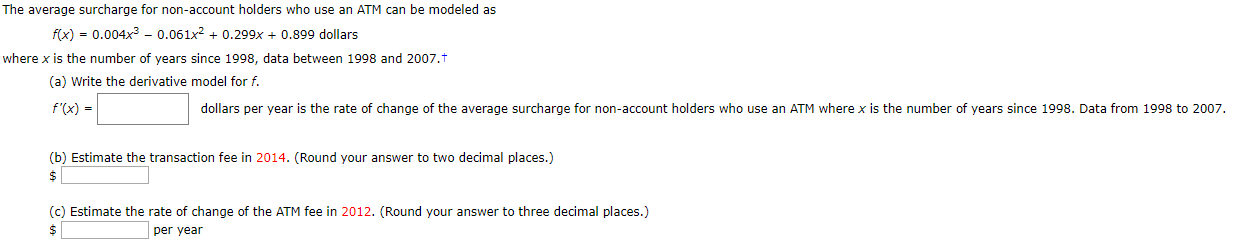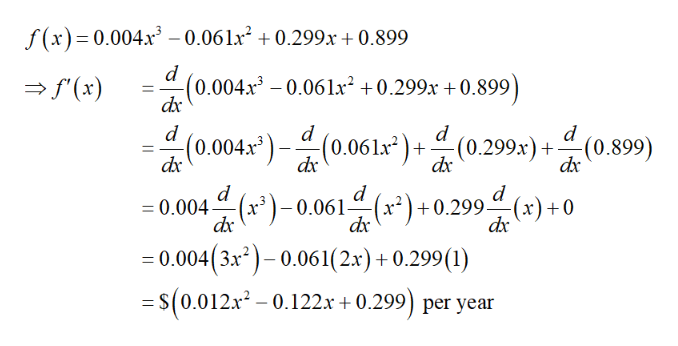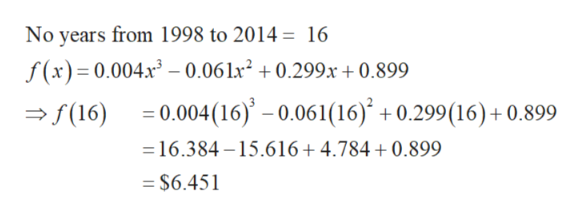# The average surcharge for non-account holders who use an ATM can be modeled asfix) = 0.004x3 - 0.061x20.299x0.899 dollarswhere x is the number of years since 1998, data between 1998 and 2007.t(a) Write the derivative model for f.f'(x)dollars per year is the rate of change of the average surcharge for non-account holders who use an ATM where x is the number of years since 1998. Data from 1998 to 2007.(b) Estimate the transaction fee in 2014. (Round your answer to two decimal places.)\$(c) Estimate the rate of change of the ATM fee in 2012. (Round your answer to three decimal places.)per year\$

Questionhelp_outlineImage TranscriptioncloseThe average surcharge for non-account holders who use an ATM can be modeled as fix) = 0.004x3 - 0.061x2 0.299x0.899 dollars where x is the number of years since 1998, data between 1998 and 2007.t (a) Write the derivative model for f. f'(x) dollars per year is the rate of change of the average surcharge for non-account holders who use an ATM where x is the number of years since 1998. Data from 1998 to 2007. (b) Estimate the transaction fee in 2014. (Round your answer to two decimal places.) \$ (c) Estimate the rate of change of the ATM fee in 2012. (Round your answer to three decimal places.) per year \$ fullscreen
check_circleExpert Solution
Step 1

(a) To find the derivative of the given function f as follows.help_outlineImage Transcriptionclosef(x) 0.004x3 - 0.061x2 0.299x +0.899 d f(x) d0.004x30.06 lx2 +0.299x +0.899 d -(0.299x)+ d (0.899) d dr0.004x "(0.061x dx d d =0.004 r)-0.061- dx d x)+0.299(x)+0 dx =0.004(3x2)-0.061(2x) +0.299 (1) = S(0.012x2-0.122.x + 0.299) per year fullscreen
Step 2

(b) To find the estimate fee ...help_outlineImage TranscriptioncloseNo years from 1998 to 2014 = 16 f(x) 0.004x3-0.06 lx2 +0.299x+ 0.899 0.004(16)' - 0.061(16) +0.299(16) + 0.899 (16) 16.384-15.616+ 4.784 + 0.899 = \$6.451 fullscreen

### Want to see the full answer?

See Solution

#### Want to see this answer and more?

Solutions are written by subject experts who are available 24/7. Questions are typically answered within 1 hour*

See Solution
*Response times may vary by subject and question
Tagged in

### Derivative